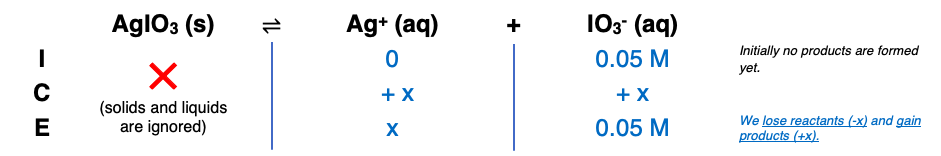# Problem: A 50.0-mL sample of 0.0413 M AgNO3(aq) is added to 50.0 mL of 0.100 M NaIO 3(aq). Calculate the [Ag+] at equilibrium in the resulting solution. [K sp for AgIO3(s) = 3.17 X 10 -8.]

###### FREE Expert Solution

Step 1

Total volume = 50.0 mL + 50.0 mL = 100 mL

= 0.05 M

Step 295% (27 ratings)###### Problem Details

A 50.0-mL sample of 0.0413 M AgNO3(aq) is added to 50.0 mL of 0.100 M NaIO 3(aq). Calculate the [Ag+] at equilibrium in the resulting solution. [K sp for AgIO3(s) = 3.17 X 10 -8.]

Frequently Asked Questions

What scientific concept do you need to know in order to solve this problem?

Our tutors have indicated that to solve this problem you will need to apply the Selective Precipitation concept. If you need more Selective Precipitation practice, you can also practice Selective Precipitation practice problems.

What professor is this problem relevant for?

Based on our data, we think this problem is relevant for Professor Chavez's class at OAKLAND.

What textbook is this problem found in?

Our data indicates that this problem or a close variation was asked in Chemistry: An Atoms First Approach - Zumdahl Atoms 1st 2nd Edition. You can also practice Chemistry: An Atoms First Approach - Zumdahl Atoms 1st 2nd Edition practice problems.